# How To Solve Physics Problems

Proper problem-solving techniques not only help guide you through the difficulties of a problem but also enable you to communicate your solution effectively to others. When working out solutions to problems in this course, please bear the following points in mind.

1. Draw as many diagrams of the situation described in the problem statement as are necessary to make the situation and your analysis clear. Often, one will suffice. On your diagrams, use symbols not values. Near the diagram, draw up a table of the givens, being sure to respect the precision and units given in the problem statement.

2. Read the entire question or problem as many times as necessary to be confident that you now precisely what is being asked in each section. Make a mental note whether the same intermediate quantity will figure in several calculations. If not, numerical values should only be substituted into completely derived algebraic expressions for the requested quantities.

3. In force problems, isolate the appropriate components of the system and sketch a force diagram for them. Put a coordinate system on each diagram. Deduce the appropriate equations of motion. In other problems, cite the appropriate laws and relations, and justify the equations you deduce where necessary. Be certain that all symbols you use have been defined, either by context or explicitly.

4. Complete the algebra using symbols; outline your formula. If no values have been provided, check your formula using dimensional analysis. If values have been provided, substitute them into the formula, being sure to respect their precision and units. Compute the requested value. Round your answer to the precision of the least precise given value upon which your result depends. In cases where a given is written as 800 km, for example, use 1, 2, or 3 significant figures based on the precision of the other given values. Be sure that the units work out. Outline the final (rounded) answer written in appropriate units. An answer of 1.0 x 107 s should often be converted to 120 days or 3.8 months to make the answer more meaningful or useful. In subsequent calculations, use unrounded values and round at the end. Most importantly, ask yourself, "Does the answer make sense?"

5. Look at your answers and ask if they make physical sense. If they don't, go back over the calculation. Check the formula to be sure that it displays the appropriate dependencies on the givens. Should the velocity increase or decrease with time? As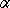goes to zero or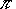/2, does your formula simplify properly? etc. Finally and most importantly, ask yourself what you have learned.

## Sample Problem

Halliday, Resnick, and Krane, 6-26, p. 125.

Object B weighs 94.0 lb and object A weighs 29.0 lb. Between object B and the plane the coefficient of static friction is 0.56 and coefficient of kinetic friction is 0.25. (a) Find the acceleration of the system if B is initially at rest. (b) Find the acceleration if B is moving up the plane. (c) What is the acceleration if B is moving down the plane? The plane is inclined by 42.0°.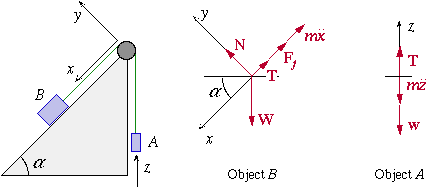```W = 94.0 lb            uk = 0.25
w = 29.0 lb            us = 0.56
[[alpha]] = 42.0deg.

```

From the force diagram for A, we get the equation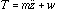From the force diagram for B, we get the pair of equations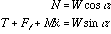.

If we assume that the string is inextensible, then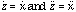. We further assume that the string and pulley are massless, and that the pulley is frictionless. Then the tension appearing the the first and third equations is the same.

(a) The frictional force must be less than or equal to the product of the normal force and the coefficient of static friction, us, for the object to remain at rest. Let us calculate u assuming the objects remain at rest to determine whether this is less than the given value. Substituting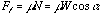in the third equation gives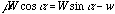,

which simplifies to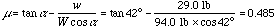.

Since u < us, the objects remain at rest.

(b) If B is initially moving up the plane, then the sense of the frictional force is opposite to that indicated in the diagram. We can solve for either case by writing the third equation as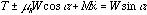,

where the upper sign corresponds to the case of motion down the plane, and the lower sign to motion up the plane. Solving for the acceleration gives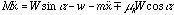,

which simplifies to.

This clearly has the right dimensions. Plugging in numbers and using g = 32.2 ft s-2 gives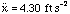for motion up the plane, and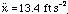for motion down the plane. These values have been rounded to 3 significant figures, in agreement with the given information. Is it reasonable that the acceleration for motion down the plane is greater? Yes, because while friction opposes the motion in both cases, gravity opposes the motion in the former case and drives it in the latter. Furthermore, based on our choice of coordinate system as indicated on the first sketch, the acceleration is down the plane, as it must be.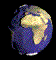Copyright © 2001 Harvey Mudd College Physics Department http://www.physics.hmc.edu/ WebMaster@Physics.hmc.edu This page was last modified on Fri, Aug 29, 1997.